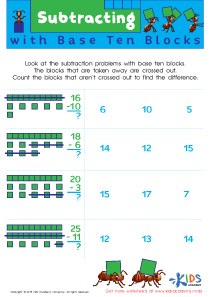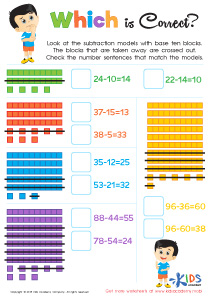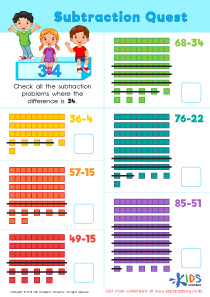# Math Lesson - Base 10 and standard Subtraction, Grade 1

• ### Activity 2 / Subtracting with Base Blocks WorksheetBase ten blocks offer little mathematicians concrete ways to understand how place value works when it comes to subtracting. With this fun and easy-to-follow worksheet, they'll look at the subtraction problems and then use the blocks that are crossed out to help them solve the subtraction problems with accuracy. When they can see equations in representation, they'll be able to work on their automaticity with number reasoning as they come across larger numbers.

• ### Activity 3 / Which Is Correct? Math WorksheetYoung mathematicians will be able to gain mathematical confidence as they use this bright and colorful worksheet to solve subtraction problems. They'll be able to use the base ten blocks representations to subtract, and then they'll look for the appropriate number sentence to match the representation of the blocks. It's great practice for developing number sense that will lead to automaticity for higher-level math problems.

• ### Activity 4 / Subtraction Quest WorksheetLet your little mathematicians take a math quest full of fun! This free and downloadable PDF worksheet will have them use base ten block representations to decide whether the difference for each problem is 34. They'll solve each problem in the quest, and then mark the equations where the difference is 34, building number sense and challenging themselves at the same time! They won't even know it's math because it'll be so much fun!

?